## Chapter 5. Building a Scene Graph

A scene graph can be a single node or a hierarchy of nodes, as shown in Figure 5-1.

Figure 5-1. Scene Graph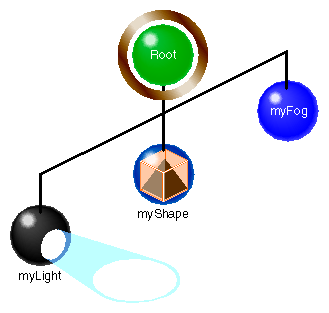The hierarchy specifies the order in which the nodes are acted upon when an action is applied to the scene graph. The hierarchy is established by the order in which the group nodes are added to the branches in a scene graph branch.

This chapter describes how to build and edit a scene graph.

This chapter includes the following sections:

## Creating Scene Graphs

The top node in a scene graph is called the root node; it must be a group-type node. Actions applied to the root node visit all of the children nodes of the root node.

 ```root->addChild(myLight); root->addChild(myShape); ```

In this simple example, the myLight node is added first to the scene graph whose root node is called root; the myShape node is added second. When a draw action is applied to the root node, either of these nodes may be evaluated first.

To complete the scene graph, you add children to any child nodes of the root node that are a group-type. You continue adding children to group-type nodes until the complete scene is encapsulated in the scene graph.

### Root Node

Most scene graphs have a root node of type csGroup. If you have a one-node scene graph, for example, a csShape node, which is a leaf node, then the root node, the only node, is a leaf node.

It is also possible to have multiple root nodes for a scene graph, as shown in Figure 5-2.

Figure 5-2. Multiple Root Nodes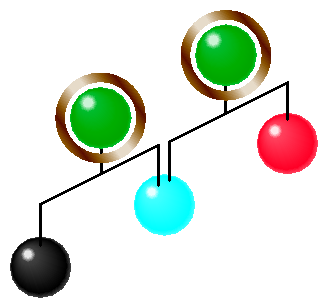### Applying Actions to Multiple Root Nodes

If a scene graph has multiple root nodes, an action applied to one of the roots would only traverse the descendants of the specific root node. While this hierarchy of nodes is legal, if your application is going to draw both scene graphs anyway, you should create a single root node common to both scene graphs. In Figure 5-2, this could be done by adding a group node above the root nodes shown, thus making them children of the single group node. The advantage to this construction is that you do not have to apply the same action repeatedly to different root nodes.

Actions applied to a root node flow (potentially) to all of the other nodes in the scene graph. Passing the action from one node to another is called traversing. As an action traverses a scene graph, variables set by the nodes in the scene graph change the graphical context, which, in turn, changes the objects in the scene according to the node values.

### Creating A Sample Scene Graph

Example 5-1 is a simple scene graph.

Example 5-1. A Simple Scene Graph

 ```// create the root node of the scene graph csGroup *root = new csGroup; // create the nodes for the scene graph csSpotLight *mySpotLight = new csSpotLight; csShape *myShape1 = new csShape; csShape *myShape2 = new csShape; // Add the nodes to the group node to create a scene graph root->addChild(mySpotLight); root->addChild(myShape1); root->addChild(myShape2); ```

In this example, a root node is created using the new directive and children nodes are added to it using the csGroup::addChild() method. The order in which the children are added to the root node is the order in which the nodes are acted upon when an action is applied to the root node.

## Diagramming Scene Graphs

Diagramming a scene graph is helpful in visualizing the structure of a Cosmo 3D application. Figure 5-3 shows a diagram representing the scene graph coded in Example 5-1.

Figure 5-3. Simple Scene GraphIn diagrams of scene graphs, circles represent nodes and lines represent the node hierarchy. The different types of circles represent the different types of nodes, for example, root is a csGroup-type node whereas myShape is a csLeaf-type node. Notice how the nodes are positioned: the three leaf nodes are children of the csGroup node and the leaf nodes appear in the same order in which they were added to the root node in Example 5-1. Remember, however, that actions may traverse these leaf nodes in any order because these leaf nodes are at the same level.

### Scene Graph Diagrams At A Glance

Diagrams of scene graphs provide an overview of the functionality of a Cosmo 3D application without the bother of delving into the complexity of the code. A diagram of a scene graph, for example, can show the number of data sets that can be rendered.

For more information about the order in which nodes are acted upon, see “The Order In Which Actions Are Passed Between Nodes”.

There are no rules for constructing a scene graph, however, it is customary to organize it in the following way:

• Reading the nodes left to right shows you the different geometries rendered in a scene graph.

• Reading the nodes from top to bottom shows you the different parts that are combined to form a larger geometry.

For example, reading horizontally, Figure 5-4 shows that the two subgraphs, Molecules and Hydrogen bonds, are separate geometries that appear together in world space.

Figure 5-4. Two Sets of Data Rendered Differently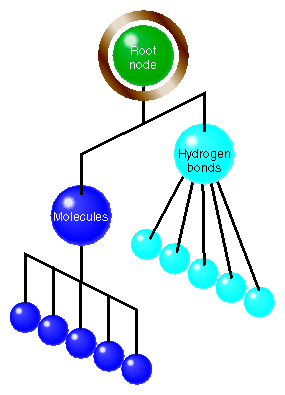In the subgraph shown in Figure 5-5, you can see that the foot, leg, and torso nodes are parts which, when rendered together, display the lower half of a body.

Figure 5-5. Torso Subgraph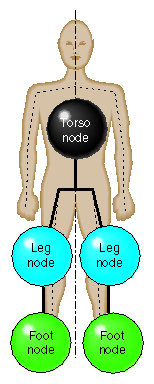Because the left leg looks different from the right leg, you need two different shape nodes. If, however, you want to display the same geometry twice, but in different locations, you can use two transformation nodes to place the same object in different locations, as shown in Figure 5-6.

Figure 5-6. Showing the Same Geometry in Two Locations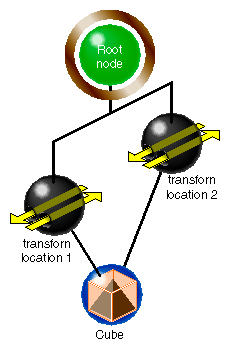In this example, the scene graph makes it easy to see that a cube is rendered in two locations by two csTransform nodes.

## Altering Scene Graphs

After using csGroup::addChild() to create a scene graph, you can use the following methods to edit it:

 ```void removeChild(int i); int  removeChild(csNode *node); int  replaceChild(csNode *old, csNode *node); void insertChild(int i, csNode *node); ```

These methods allow you to remove, replace, or insert a child node, respectively. For example, to insert a node between two children, use the insertChild() method:

 ```csShape *myShape = new csShape; root->insertChild(2, myShape); ```

The children nodes are numbered starting with 0. The “2” in the argument of insertChild() specifies that the myShape node should be inserted in the scene graph as the number two node.Note: Although leaf nodes attached to the same group node can be acted upon in any order, the first node added to a group node is always node zero, the second node added to the root node in the code is node one, and so forth.

csGroup also supplies the following methods for finding the number of a node in a scene graph, returning the number of children in a scene graph, and setting the number of nodes in a group, respectively.

 ```int  searchChild(csNode *node); int  getChildCount(); void setChildCount(int count); ```

In general, you use the searchChild() method to return the number of a node so you can perform other functions on or around it, such as replacing it.

Example 5-2 shows a portion of vrml.cxx. The example illustrates how to load a VRML scene graph using vlDB::readFile().

 ```csGroup *vrml = new csGroup; for (int i=1; iaddChild((csNode*)v); } else printf(“Read %s was bad\n”, argv[i]); } new csWindow(“vrml”); ```

## Saving Scene Graphs

The data in the scene graph database is not necessarily static. You might, therefore, need to save scene graph data into a file. To do so, you use the following method:

 ```csGlobal::storeFile(NameOfFile, *dataStructure); ```

where NameOfFile is the name of the file where you want to store the data and dataStructure is the Cosmo 3D in-memory data structure to store. The method returns TRUE if the file is stored successfully, FALSE otherwise.Note: storeFile() is used with Cosmo 3D-only applications. If you are writing an Optimizer application, do not use storeFile().

## Troubleshooting Scene Graph Construction

A common mistake in Cosmo 3D applications is forgetting to include a csLight or csCamera node. The csDrawAction::setCamera() method specifies the camera and points it at the shapes in the scene graph.

For more information about csLight or csCamera, see Chapter 8, “Lighting and Fog” and Chapter 9, “Viewing the Scene,” respectively.

Another common error in Cosmo 3D applications is pointing the camera in the wrong direction in which case the camera may produce a blank image.